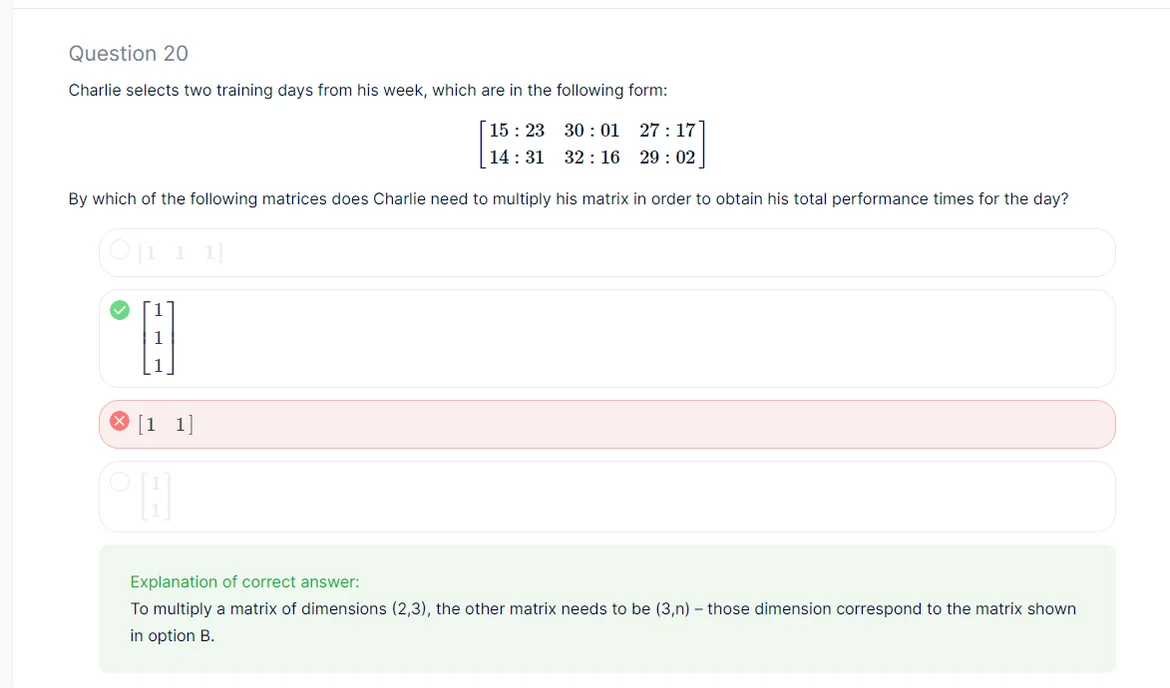Posted on:

30 Oct 2022

0

# Course Exam - Q20

I think in this question, the answer is relative to the order we are multiplying the two matrices
In other words, b is correct if considered to multiply the original matrix (2,3) * b-answer (3,1)
while c can be correct if we considered the multiplication to be c-answer (1,2) * original matrix (2,3)

Is it correct?